# Excel cell conversion tutorial ŌĆō Convert units, text and number, currencies, time zones, etc

When dealing with data in Excel, there are instances when you may find that you need to do certain data conversions on cells, such as converting units, converting currencies, converting time zones, and so on.

## Convert Units

In most cases, when we need to convert a number from one unit of measure to another, we will utilize the CONVERT function. However, the CONVERT function will not operate properly for some of the unit conversions that you attempt.

Step 1

You may use the CONVERT function to convert the inches specified in the range to the corresponding values in feet, centimeters, and millimeters individually.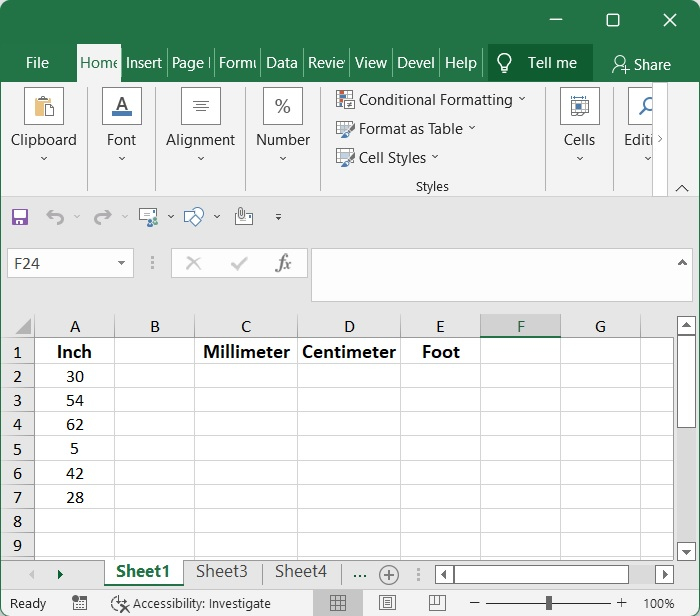Step 2

There is a diverse selection of units for measuring. To see the units that belong to each category and that may be used with the "from unit" and "to unit" parameters of the CONVERT function, type "from unit" and "to unit" respectively.

=CONVERT(number, from_unit, to_unit)


Where,

• number ŌłÆ The numerical value that has to be converted.

• from_unit ŌłÆ a number's initial measurement unit.

• to_unit ŌłÆ The unit that the "number" will be converted to

### Inch to mm Conversion

Choose a cell (let's say C2 for this example), then either paste or type the formula that is provided below into that cell, and then hit the Enter key. First, select the cell that contains this result, and then use the AutoFill Handle to move it downwards. This will bring up the remaining results.

=CONVERT(A2,"in","mm")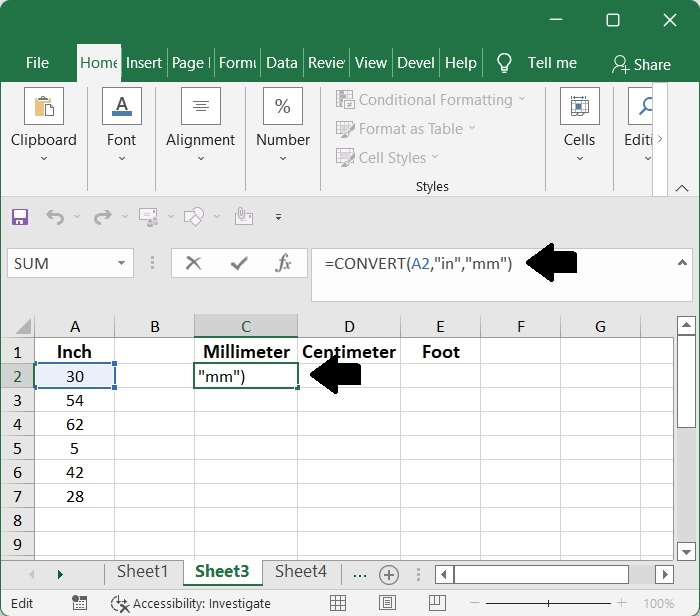Step 3

Choose a cell (let's say D2 for this example), then either paste or type the formula that is provided below into that cell, and then hit the Enter key. First, select the cell that contains this result, and then use the AutoFill Handle to move it downwards. This will bring up the remaining results.

=CONVERT(A2,"in","cm")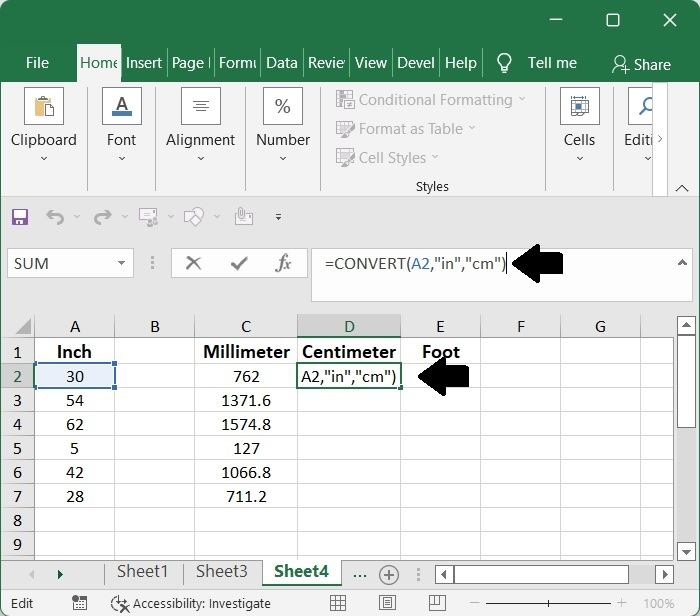Step 4

Choose a cell (let's say E2 for this example), then either paste or type the formula that is provided below into that cell, and then hit the Enter key. First, select the cell that contains this result, and then use the AutoFill Handle to move it downwards. This will bring up the remaining results.

=CONVERT(A2,"in","ft")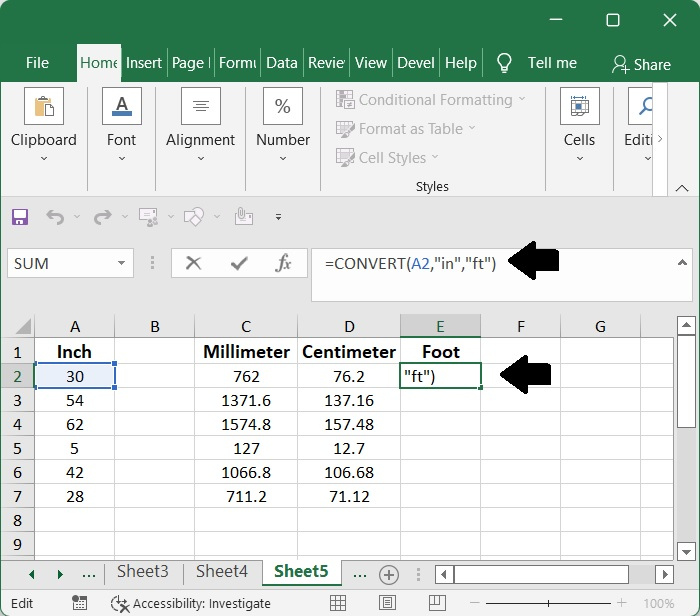## Text and Number

The following user-defined function might be of use to you in Excel if you want to show numbers as words in the English language.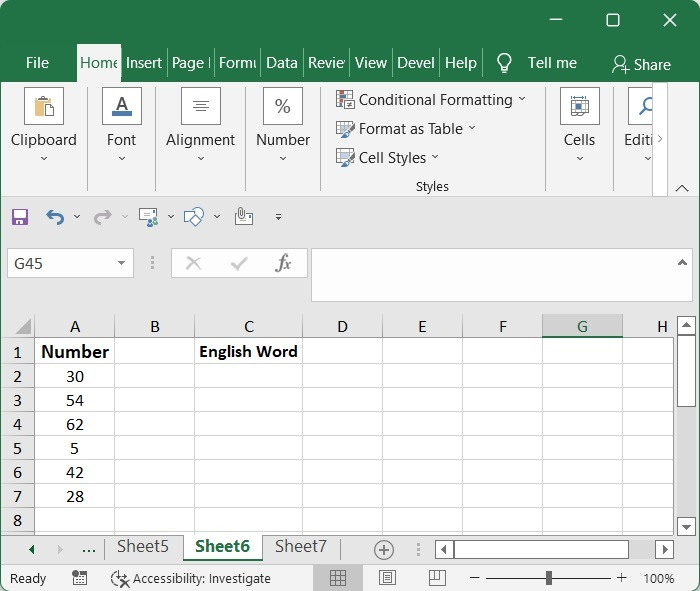Step 1

To launch the Microsoft Visual Basic for Applications window, press and hold the Alt key while simultaneously pressing the F11 key. or go to Developer menu and select Visual Basic.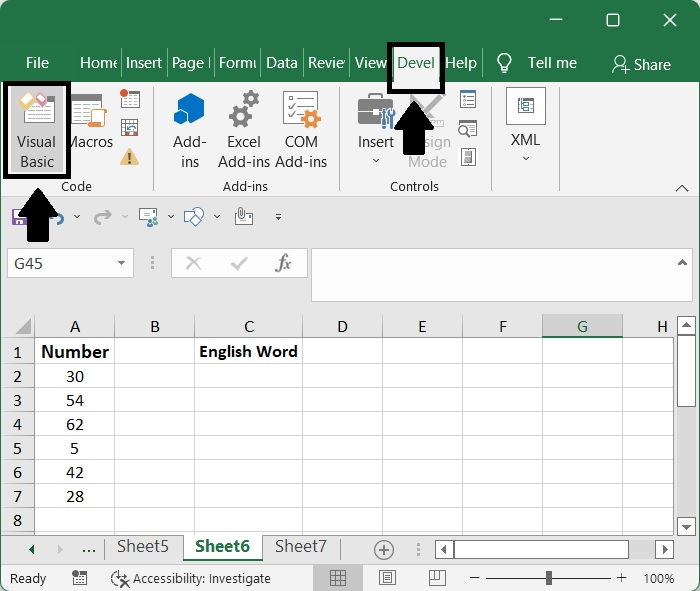After that, it opens up for Microsoft Visual Basic for Applications. Then go to Insert and select Module.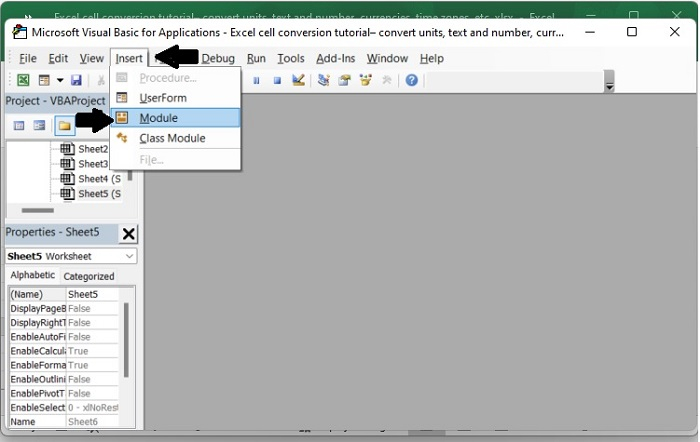And copy and paste the following VBA code.

Function NumberstoWords(ByVal MyNumber)
'Update by Extendoffice 20220516
Dim xStr As String
Dim xFNum As Integer
Dim xStrPoint
Dim xStrNumber
Dim xPoint As String
Dim xNumber As String
Dim xP() As Variant
Dim xDP
Dim xCnt As Integer
Dim xResult, xT As String
Dim xLen As Integer
On Error Resume Next
xP = Array("", "Thousand ", "Million ", "Billion ", "Trillion ", " ", " ", " ", " ")
xNumber = Trim(Str(MyNumber))
xDP = InStr(xNumber, ".")
xPoint = ""
xStrNumber = ""
If xDP > 0 Then
xPoint = " point "
xStr = Mid(xNumber, xDP + 1)
xStrPoint = Left(xStr, Len(xNumber) - xDP)
For xFNum = 1 To Len(xStrPoint)
xStr = Mid(xStrPoint, xFNum, 1)
xPoint = xPoint & GetDigits(xStr) & " "
Next xFNum
xNumber = Trim(Left(xNumber, xDP - 1))
End If
xCnt = 0
xResult = ""
xT = ""
xLen = 0
xLen = Int(Len(Str(xNumber)) / 3)
If (Len(Str(xNumber)) Mod 3) = 0 Then xLen = xLen - 1
Do While xNumber <> ""
If xLen = xCnt Then
xT = GetHundredsDigits(Right(xNumber, 3), False)
Else
If xCnt = 0 Then
xT = GetHundredsDigits(Right(xNumber, 3), True)
Else
xT = GetHundredsDigits(Right(xNumber, 3), False)
End If
End If
If xT <> "" Then
xResult = xT & xP(xCnt) & xResult
End If
If Len(xNumber) > 3 Then
xNumber = Left(xNumber, Len(xNumber) - 3)
Else
xNumber = ""
End If
xCnt = xCnt + 1
Loop
xResult = xResult & xPoint
NumberstoWords = xResult
End Function
Function GetHundredsDigits(xHDgt, xB As Boolean)
Dim xRStr As String
Dim xStrNum As String
Dim xStr As String
Dim xI As Integer
Dim xBB As Boolean
xStrNum = xHDgt
xRStr = ""
On Error Resume Next
xBB = True
If Val(xStrNum) = 0 Then Exit Function
xStrNum = Right("000" & xStrNum, 3)
xStr = Mid(xStrNum, 1, 1)
If xStr <> "0" Then
xRStr = GetDigits(Mid(xStrNum, 1, 1)) & "Hundred "
Else
If xB Then
xRStr = "and "
xBB = False
Else
xRStr = " "
xBB = False
End If
End If
If Mid(xStrNum, 2, 2) <> "00" Then
xRStr = xRStr & GetTenDigits(Mid(xStrNum, 2, 2), xBB)
End If
GetHundredsDigits = xRStr
End Function
Function GetTenDigits(xTDgt, xB As Boolean)
Dim xStr As String
Dim xI As Integer
Dim xArr_1() As Variant
Dim xArr_2() As Variant
Dim xT As Boolean
xArr_1 = Array("Ten ", "Eleven ", "Twelve ", "Thirteen ", "Fourteen ", "Fifteen ", "Sixteen ", "Seventeen ", "Eighteen
", "Nineteen ")
xArr_2 = Array("", "", "Twenty ", "Thirty ", "Forty ", "Fifty ", "Sixty ", "Seventy ", "Eighty ", "Ninety ")
xStr = ""
xT = True
On Error Resume Next
If Val(Left(xTDgt, 1)) = 1 Then
xI = Val(Right(xTDgt, 1))
If xB Then xStr = "and "
xStr = xStr & xArr_1(xI)
Else
xI = Val(Left(xTDgt, 1))
If Val(Left(xTDgt, 1)) > 1 Then
If xB Then xStr = "and "
xStr = xStr & xArr_2(Val(Left(xTDgt, 1)))
xT = False
End If
If xStr = "" Then
If xB Then
xStr = "and "
End If
End If
If Right(xTDgt, 1) <> "0" Then
xStr = xStr & GetDigits(Right(xTDgt, 1))
End If
End If
GetTenDigits = xStr
End Function
Function GetDigits(xDgt)
Dim xStr As String
Dim xArr_1() As Variant
xArr_1 = Array("Zero ", "One ", "Two ", "Three ", "Four ", "Five ", "Six ", "Seven ", "Eight ", "Nine ")
xStr = ""
On Error Resume Next
xStr = xArr_1(Val(xDgt))
GetDigits = xStr
End Function


See the screenshot below.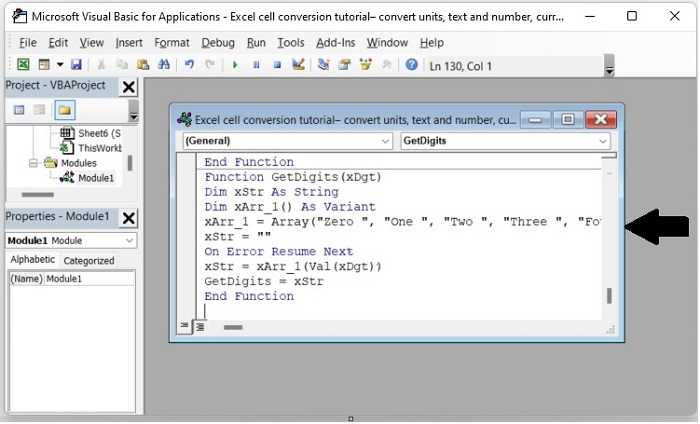Step 2

Choose a blank cell, in this example C2. Press Enter after entering the following formula ŌłÆ

=NumberstoWords(C2)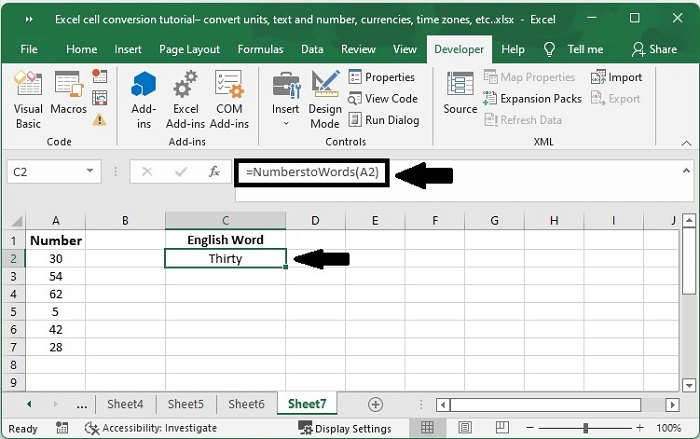Step 3

Drag the AutoFill Handle of this result cell down to access the other results after selecting this cell.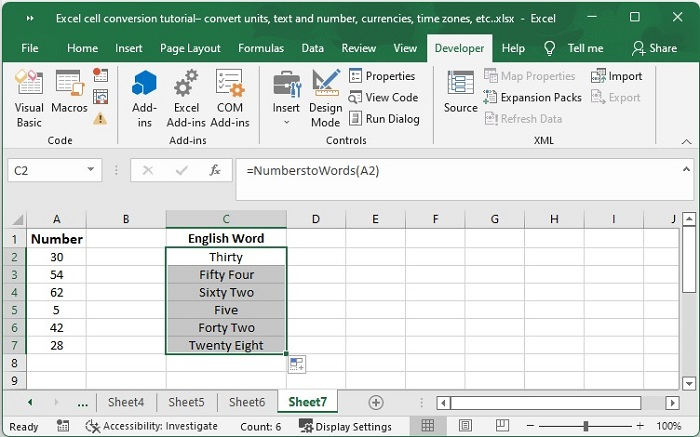## Conclusion

In this tutorial, we explained how you can convert units, text and numbers in Excel.